Sorting - linked list: there are many algorithms to sort a linked list like the bubble sort, merge sort, etc.

so today we are going to sort a linked list by exchanging the values of nodes using the bubble sort algorithm.

## Sorting a linked list using the bubble sort algorithm

In a bubble to sort a linked list that has n items, we need to iterate over the list n-1 times. like in the example we have a list of 5 items then we need to iterate over the list for 4 times.

to sort a linked list first we need three references p, q, and end. in the first iteration, we set the end variable to None. and in every iteration, we set the p variable to the first node of the list and the q variable to the second node of the list.if in the iteration the value of the linked part of node p is equal to the end then we break the iteration and continue to the next iteration.

if the info part of node p is greater than the info part of node q then we swap the values of node p and q otherwise we refer p and q to the next node of the list.
so in the given example list, the value of node p is not greater than the value of the q node so we refer p and q to the next node of the list.here also the value of node p is not greater than the value of node q so we go to the next nodes.here the value of node p is greater than the value of node q so we swap them.next, we go to the next nodes here the value of node p is not greater than the value of node q so we don't swap them.now we go to the next nodes of the list. here the value of the linked part of node p is equal to the end variable. so here the first iteration ends.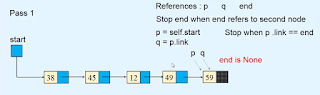now after iteration one ends, the last node of the list becomes sorted so we refer the end variable to the last node of the list.now the second iteration starts and in the second iteration, we refer the variables p and q to the first and second node of the list and swap values of nodes p and q if the values of node p are greater than the value of node q.

this condition will run till the linked part of node p does not become equal to the end variable. as you see in the images given below.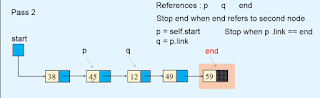and after every iteration, we update the value of the variable end. because if we iterate the list over two times then the last two nodes of the list become sorted. so we don't need to check them again.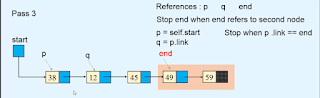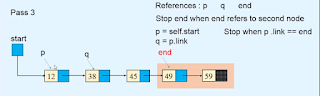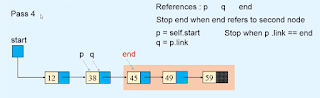so after performing the n-1 iterations over the linked list. where n is the size of the list. now our list becomes sorted.

```def bubble_sort_exdata(self):

end = None
p = self.start
if p.info > q.info:
p.info, q.info = q.info, p.info
end = p```

#### Python program for traversing the linked list.

```class Node:

def __init__(self, value):
self.info = value

def __init__(self):
self.start = None
def display_list(self):
if self.start is None:
print("List is empty")
return        else:
print("List is :   ")
p = self.start
while p is not None:
print(p.info, " ", end=' ')
print()

def count_nodes(self):
p = self.start
n = 0        while p is not None:
n += 1            p = p.link
print("Number of nodes in the list = ", n)

def search(self):
position = 1        p = self.start
while p is not None:
if p.info == x:
print(x, " is at position ", position)
return True            position += 1            p = p.link
else:
return False
def insert_in_beginning(self, data):
temp = Node(data)
self.start = temp

def insert_at_end(self, data):
temp = Node(data)
if self.start is None:
self.start = temp
return
p = self.start

def create_list(self):
n = int(input("Enter the number of nodes  :  "))
if n == 0:
return        for i in range(n):
data = int(input("Enter the element to be inserted : "))
self.insert_at_end(data)

def insert_after(self, data, x):
p = self.start
while p is not None:
if p.info == x:

if p is None:
print(x, "not present in the list")
else:
temp = Node(data)

def insert_before(self, data, x):
# If list is empty        if self.start is None:
print("List is empty")
return
if x == self.start.info:
temp = Node(data)
self.start = temp
return

p = self.start

print(x, " not present in the list")
else:
temp = Node(data)

def insert_at_position(self, data, k):
if k == 1:
temp = Node(data)
self.start = temp
return
p = self.start
i = 1        while i < k - 1 and p is not None:
i += 1
if p is None:
print("You can insert only upto position", i)
else:
temp = Node(data)

def delete_node(self, x):

if self.start is None:
print("List is empty")

if self.start.info == x:
return
p = self.start

print("Element ", x , "not in list")
else:

def delete_first_node(self):
if self.start is None:

def delete_last_node(self):

if self.start is None:
return
self.start = None            return
p = self.start
def reverse_list(self):

prev = None        p = self.start
while p is not None:
prev = p
p = next
self.start = prev

def bubble_sort_exdata(self):

end = None
p = self.start
if p.info > q.info:
p.info, q.info = q.info, p.info
end = p

end = None
r = p = self.start
if p.info > q.info :
if p != self.start:
else:
self.start = q
p,q = q,p
r = p
end = p

def has_cycle(self):
if self.find_cycle() is None:
return False        else:
return True
def find_cycle(self):
if self.start is None or self.start.link is None:
return None
slowR = self.start
fastR = self.start

while fastR is not None and fastR.link is not None:
if slowR == fastR:
return slowR
return None
def remove_cycle(self):
c = self.find_cycle()
if c is None:
return        print("Node at which the cycle was detected is ", c.info)

p = c
q = c
len_cycle = 0
while True:
if p == q:
break        print("Length of cycle is :", len_cycle)

len_rem_list = 0        p = self.start
while p != q:

print("Number of nodes not included in the cycle are : ", len_rem_list)
length_list = len_cycle + len_rem_list
print("Length of the list is : ", length_list)

p = self.start
for i in range(length_list-1):

def insert_cycle(self, x):
if self.start is None:
return        p = self.start
px = None        prev = None
while p is not None:
if p.info == x:
px = p
prev = p

if px is not None:
else:
print(x, " not present in list")

def merge2(self, list2):
merge_list.start = self._merge2(self.start, list2.start)
return merge_list

def _merge2(self, p1, p2):
if p1.info <= p2.info:
startM = p1
else:
startM = p2
pM = startM

while p1 is not None and p2 is not None:
if p1.info <= p2.info:
else:

if p1 is None:
else:

return startM

def merge_sort(self):
self.start = self._merge_sort_rec(self.start)

def _merge_sort_rec(self, list_start):

if list_start is None or list_start.link is None:
return list_start

start1 = list_start
start2 = self._divide_list(list_start)
start1 = self._merge_sort_rec(start1)
start2 = self._merge_sort_rec(start2)
startM = self._merge2(start1, start2)

return startM

def _divide_list(self, p):
while q is not None and q.link is not None:

##########################

list.create_list()

while True:
print("1. Display list")
print("2. Count the number of nodes")
print("3. Search for an element")
print("4. Insert in empty list/insert in beginning of the list")
print("5. Insert a node at the end of the list")
print("6. Insert a node after a specified node")
print("7. Insert a node before a specified node")
print("8. Insert a node at a given position")
print("9. Delete first node")
print("10. Delete last node")
print("11. Delete any node")
print("12. Reverse the list")
print("13. Bubble sort by exchanging data")
print("14. Bubble sort by exchanging links")
print("15. Merge sort")
print("16. Insert Cycle")
print("17. Detect Cycle")
print("18. Remove Cycle")
print("19. Quite")

if option == 1:
list.display_list()
elif option == 2:
list.count_nodes()
elif option == 3:
data = int(input("Enter the element to be searched : "))
list.search(data)
elif option == 4:
data = int(input("Enter the element to be inserted : "))
list.insert_in_beginning(data)
elif option == 5:
data = int(input("Enter the element to be inserted : "))
list.insert_at_end(data)
elif option == 6:
data = int(input("Enter the element to be inserted : "))
x = int(input("Enter the element after which to insert : "))
list.insert_after(data, x)
elif option == 7:
data = int(input("Enter the element to be inserted : "))
x = int(input("Enter the element before which to insert : "))
list.insert_before(data, x)
elif option == 8:
data = int(input("Enter the element to be inserted : "))
k = int(input("Enter the position at which to insert : "))
list.insert_at_position(data, k)
elif option == 9:
list.delete_first_node()
elif option == 10:
list.delete_last_node()
elif option == 11:
data = int(input("Enter the element to be deleted : "))
list.delete_node(data)
elif option == 12:
list.reverse_list()
elif option == 13:
list.bubble_sort_exdata()
elif option == 14:
elif option == 15:
list.merge_sort()
elif option == 16:
data = int(input("Enter the element at which the cycle has to be inserted : "))
list.insert_cycle(data)
elif option == 17:
if list.has_cycle():
print("List has a cycle")
else:
print("List does not have a cycle")
elif option == 18:
list.remove_cycle()
elif option == 19:
break    else:
print("Wrong option")
print()```